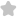If a, b care in A.P. and a, b, care...
Question# If a, b care in A.P. and a, b, care in G.P. such that a JEE/Engineering Exams Maths SolutionShare804.0 (1 ratings)of ( 6 t quad b=a+d, ) given ( a+b+c=frac{3}{4} ) ( a=b-d ) ( & quad c=b+d ) ( Rightarrow a+b+c=frac{3}{4} Rightarrow 3 b=frac{3}{4} Rightarrow b=frac{1}{4} ) ( therefore ) the number are is ( a, b, c ) in ( A cdot P ). ( =frac{1}{4}-d, frac{1}{4}, frac{1}{4}+d(d>0 text { as } b804.0 (1 ratings) Rate SolutionShareQuick and Stepwise Solutions Just click and SendOVER 20 LAKH QUESTIONS ANSWERED Download App for Free